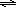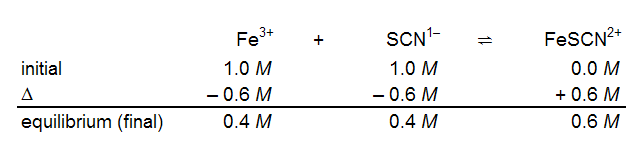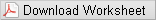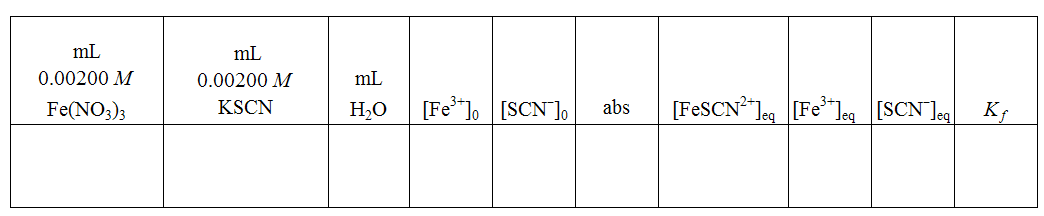# Lab Investigation 5 - What Is the Formation Constant for FeSCN2+?

## Guiding Question

What is the formation constant for the FeSCN2+ system?

## Introduction

A typical chemical equation has the following form:
( 1 )
WaTeX parsing error.
This form of the equation assumes that the reaction proceeds completely to products. In practice, many reactions do not proceed to completion. If we measure the concentration of a reactant, it eventually reaches a value that does not change further over time. If we measure the concentration of a product, it reaches a constant value short of that predicted by the theoretical yield calculation. In these cases, we say that the reaction has reached equilibrium. We write the chemical reaction using equilibrium arrows instead of a single arrow:
( 2 )
aA + bBcC + dD.

At equilibrium, the rates of the forward and reverse reactions are equal and, unless equilibrium is disturbed (stressed), no changes in reactant or product concentrations will be measured. The equilibrium arrows, one of which points in each direction, reinforce this idea. At equilibrium, the molar concentrations of products and reactants will be fixed in a given ratio. This ratio is the equilibrium constant,
Keq
which is determined by substituting molar concentrations (indicated by the square brackets) into the equilibrium constant equation. The general form of this equation is:
( 3 )
Keq =
 [C]c[D]d [A]a[B]b
Reactants mixed in arbitrary concentrations will react until the ratio of the concentrations reaches the value of the equilibrium constant according to equation 3 . The value of
Keq
varies with temperature; therefore, the temperature at which the equilibrium constant was determined must be referenced.
In this laboratory experiment, a combination of solution chemistry, stoichiometry and spectrophotometric analysis will be used to determine the equilibrium constant for a reaction between iron(III) ion (Fe3+) and thiocyanate ion (SCN). In acidic solution, these ions form a blood-red complex ion as shown in equation 4 :
( 4 )
Fe3+(aq) + SCN(aq)FeSCN2+(aq).

The equilibrium constant for equation 4 can be expressed using the concentrations of the three components:
( 5 )
K =
 [FeSCN2+] [Fe3+][SCN−]
In order to calculate the equilibrium constant, one must simultaneously determine the concentrations of all three of the components. In this experiment, you will measure the concentration of FeSCN2+ at equilibrium by measuring its absorbance at 470 nm. Since Fe3+ and SCN do not absorb light at this wavelength, they do not interfere with the measurements. If you know the initial (before equilibrium) concentrations of Fe3+ and SCN, you can use a reaction table to calculate the equilibrium concentrations of these two ions at equilibrium. For example, you might initially mix equal volumes of 2.0 M
Fe3+
and 2.0 M
SCN
The term "initial concentration" can be confusing. Even though the reaction appears to go instantaneously upon mixing the reactants, the "initial concentrations" in the reaction table are those after dilution has been taken into consideration but before any reaction occurs. Thus, the initial line in the reaction table for mixing equal volumes of 2.0 M
Fe3+
and 2.0 M
SCN
should have entries of 1.0 M under
Fe3+
and
SCN
due to dilution. The initial concentration of
FeSCN2+
is 0.0 M. In our example, you might measure an equilibrium (final) concentration of 0.6 M
FeSCN2+
With the final concentration of the product, you can determine the change in product concentration and, therefore, the changes in the reactant concentrations. The reaction table is shown below.In this experiment, 0.2 M HNO3 serves as the solvent. The acid adds a large (compared to the reactants) amount of H+. This prevents side reactions such as the formation of FeOH2+, a brownish species that can affect the results. The acid concentration is high enough that it is not affected by the reaction and remains constant at 0.2 M. You will prepare six standard solutions of
FeSCN2+
to calibrate a spectrophotometer. A fair question is
"How do I know the concentration of FeSCN2+ in my standard solutions if it is in equilibrium with Fe3+ and SCN?" In the standard solutions, the concentration of Fe3+ is much higher than that of SCN. This forces the equilibrium as far to the right (toward FeSCN2+) as possible. Therefore, the concentration of FeSCN2+ in a standard solution will be very nearly equal to the initial concentration of SCN used in preparing it. The absorbance measurement at 470 nm will correlate to the concentration of complex ion, and an accurate calibration curve (Beer's law plot) can be obtained. Recall that the calibration curve gives you a relationship between the concentration of a species in solution and its absorbance at a given wavelength: (A = εlc). Using the linear regression of the calibration curve in Part A, you will determine the concentration of FeSCN2+ ion in each of five equilibrium mixtures in Part B. An equilibrium constant can then be determined for each mixture; the average should be the equilibrium constant value for the formation of the FeSCN2+ ion.
In Part A of this experiment, you will prepare FeSCN2+ solutions of known concentrations, measure their absorbances at 470 nm, and produce a calibration curve. In Part B, you will make equilibrium mixtures of Fe3+, SCN, and FeSCN2+. You will determine the concentration of FeSCN2+ from its absorbance at 470 nm and your calibration curve from Part A. Then, using reaction tables, you will calculate the equilibrium concentrations of Fe3+ and SCN, and determine the equilibrium constant for the formation of FeSCN2+.

## Goals

As you complete this investigation you will:
• 1
Design a procedure for measuring the concentration of FeSCN2+ from the color intensity of a solution.
• 2
Design and carry out a procedure to measure the equilibrium constant for a complex ion formation reaction.

## Materials Available For Use

• 0.100 M Fe(NO3)3 in 0.2 M HNO3
• 5.00 × 10−4 M NaSCN in 0.2 M HNO3
• 0.00200 M Fe(NO3)3 in 0.2 M HNO3
• 0.00200 M NaSCN in 0.2 M HNO3
• Pipettors and tips
• Vernier LabPro system with colorimeter
• Cuvettes
• Kimwipes

## Safety Precautions

Caution:
Wear goggles at all times.
Caution:
Nitric acid is listed as a corrosive. Corrosives can attack the skin and cause permanent damage to the eyes. Nitric acid and iron(III) nitrate are listed as oxidants. Sodium thiocyanate is listed as toxic and an irritant. With the exception of nitric acid, the concentrations of all these materials are quite low, however. If you spill any of these chemicals on skin or clothing, flush the area immediately with water.

## Waste Disposal

All of the solutions prepared in this experiment, as well as excess NaSCN solution, should be discarded in the waste container. You may wish to have a waste beaker in your work area to collect waste while you are doing the experiment. Make sure it is labeled. Always remember not to overfill the waste bottle. If your waste bottle is full, please alert your lab instructor.

## Procedure

Please print the worksheet for this lab. You will need this sheet to record your data.### Part A: Preparation of Standard Solutions and Beer's Law Plot

Because the FeSCN2+ complex ion is a colored species, a device that measures light absorbance can generate data for a Beer's law calibration curve. A plot of absorbance versus concentration for a set of solutions will have a linear range that can then be used to find concentration given absorbance.

### Part B: Finding the Formation Constant (Kf)

Once you have generated a calibration curve for the absorbance of the FeSCN2+ complex ion, mix four or five combinations of Fe3+ and SCN. Use varying amounts of 0.00200 M KSCN and 0.00200 M Fe(NO3)3 for each solution and make the total volume of each solution 6.00 mL. As a point of reference all groups should prepare one reaction mixture with 3.00 mL Fe3+ solution and 3.00 mL SCN solution with no added water. Determine the absorbance of the FeSCN2+ complex ion after equilibrium is established in each solution. (Equilibrium is established within a few seconds after the reactants are mixed.) Since neither Fe3+ nor SCN is in large excess, the
[FeSCN2+]eq
cannot be calculated based on initial concentrations; it must be determined using the calibration curve once the reaction is at equilibrium. Once that concentration is known, you should be able to calculate the concentrations of the other ions at equilibrium. Use the equilibrium concentrations to determine the value of
Kf
for this system.
There is a post-lab assignment in WebAssign that will enable you to check your calculations.

## Interactive Poster Session

Once your group has completed work, prepare a whiteboard that you can use to share and justify your ideas. See the handout provided for details on this process.

## Report

Once you have completed your research, you will need to prepare an investigation report that consists of three sections. This report may require more than 2 pages with data tables. This report must be typed and any diagrams, figures, or tables should be embedded into the document.
• Section 1: What concept were you investigating? Relate this concept to the guiding question. What is the formation constant for the FeSCN2+ system? Discuss the relationship of equilibrium constant and LeChatelier's principle to the guiding question.
• Section 2: How did you go about your work and why did you conduct your investigation in this way? It is important that you explain the importance of the concentration of the reactants in creating the calibration curve.
• Section 3: What is your argument? Report an average
Kf ± SD
and justify any data you throw out with a 4D test. Show one sample calculation of initial concentrations of Fe3+ and SCN from dilution and equilibrium concentrations of FeSCN2+, using absorbance; then, show one ICE chart calculation for the equilibrium concentrations of Fe3+, and SCN and the related calculation of
Kf
• This third section is where you not only present your data, but use the values you obtain as evidence in your reasoning. Statements like, "see data table for values" are not acceptable!
Remember: An argument is not just an answer to the question. It is a claim or conclusion supported by evidence with a rationale for why the evidence supports the claim or conclusion.

## Sample Data Table